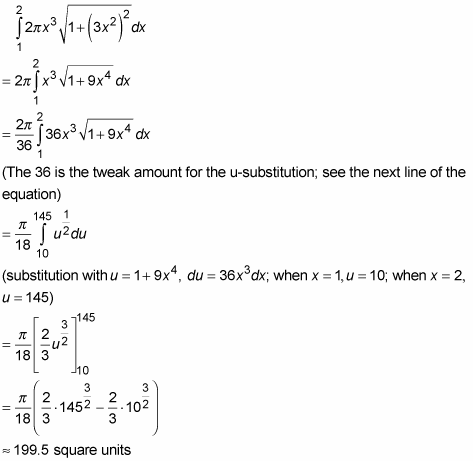##### Geometry: 1001 Practice Problems For Dummies (+ Free Online Practice)A surface of revolution is a three-dimensional surface with circular cross sections, like a vase or a bell or a wine bottle. For these problems, you divide the surface into narrow circular bands, figure the surface area of a representative band, and then just add up the areas of all the bands to get the total surface area. The following figure shows such a shape with a representative band.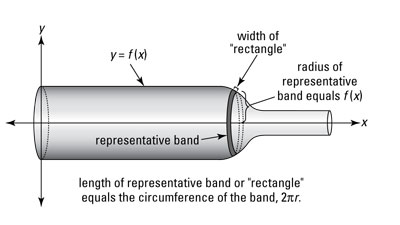What’s the surface area of a representative band? Well, if you cut the band and unroll it, you get sort of a long, narrow rectangle whose area, of course, is length times width.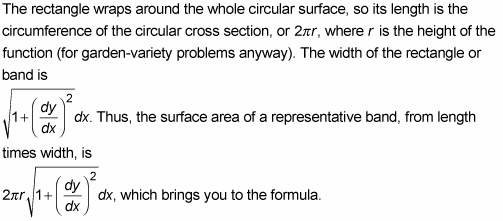Surface of Revolution: A surface generated by revolving a function, y = f (x), about an axis has a surface area — between a and b — given by the following integral: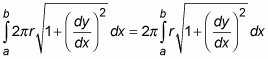By the way, in the above explanation, you might be wondering why the width of the rectangular band is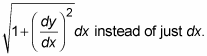It’s because the little band width is slanted instead of horizontal (in which case it would be just dx). The fact that it’s slanted makes it work like the hypotenuse of a little right triangle. The fancy-looking expression for the width of the band comes from working out the length of this hypotenuse with the Pythagorean Theorem. That should make you feel a lot better!

If the axis of revolution is the x-axis, r will equal f (x) — as shown in the above figure. If the axis of revolution is some other line, like y = 5, it’s a bit more complicated — something to look forward to.

Now try a problem: What’s the surface area — between x = 1 and x = 2 — of the surface generated by revolving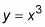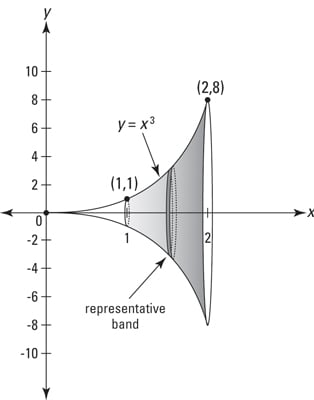A surface of revolution — this one’s shaped sort of like the end of a trumpet.
1. Take the derivative of your function.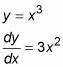Now you can finish the problem by just plugging everything into the formula, but you should do it step by step to reinforce the idea that whenever you integrate, you write down a representative little bit of something — that’s the integrand — then you add up all the little bits by integrating.

2. Figure the surface area of a representative narrow band.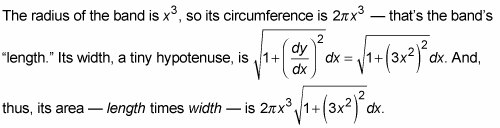3. Add up the areas of all the bands from 1 to 2 by integrating.